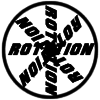#### You may also like### 8 Methods for Three by One

This problem in geometry has been solved in no less than EIGHT ways by a pair of students. How would you solve it? How many of their solutions can you follow? How are they the same or different? Which do you like best?### Rots and Refs

Follow hints using a little coordinate geometry, plane geometry and trig to see how matrices are used to work on transformations of the plane.### Reflect Again

Follow hints to investigate the matrix which gives a reflection of the plane in the line y=tanx. Show that the combination of two reflections in intersecting lines is a rotation.

# Nine Eigen

##### Age 16 to 18Challenge Level

This problem involves the action of matrices on vectors in three dimensions. The first few questions look at fixed vector directions; the latter questions look at fixed vectors. As you consider each point, make use of geometric or algebraic arguments as appropriate. Draw diagrams and construct particular examples of matrices and vectors if needed. If there is no definitive answer to a given part, try to give examples of when the question posed is or is not true.

In the questions below: $R, S$ are rotation matrices; $P, Q$ are reflection matrices; $M$ is neither a rotation nor a reflection.
1. Which of the different types of matrices can leave no vector directions fixed?
2. Which of the different types of matrices can leave exactly one vector direction fixed?
3. Which of the different types of matrices can leave more than one vector direction fixed?
4. Is it ever the case that $RS$ can leave a vector invariant?
5. Is it ever the case that $PQ$ can leave a vector invariant?
6. Is it ever the case that $M$ will leave the direction of a vector invariant?
7. Can a matrix with determinant zero leave a vector fixed?
8. Can a matrix with determinant greater than $1$ leave a vector fixed?
9. Can a matrix leave exactly two vectors fixed?